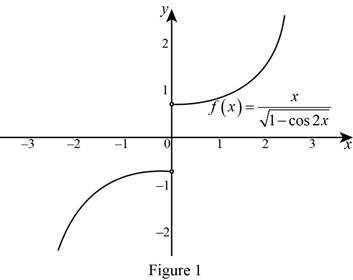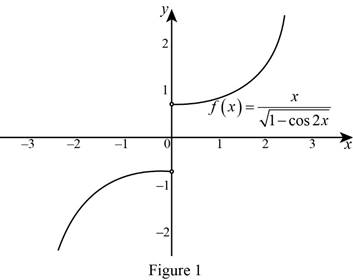# The graph of f ( x ) = x 1 − cos 2 x and describe the discontinuity at 0.### Single Variable Calculus: Concepts...

4th Edition
James Stewart
Publisher: Cengage Learning
ISBN: 9781337687805### Single Variable Calculus: Concepts...

4th Edition
James Stewart
Publisher: Cengage Learning
ISBN: 9781337687805

#### Solutions

Chapter 3.3, Problem 50E

(a)

To determine

## To sketch: The graph of f(x)=x1−cos2x and describe the discontinuity at 0.

Expert Solution

### Explanation of Solution

Definition used:

If the left hand limit of f(x) and the right hand limit of f(x) exists but are not equal, then the function f(x) said to be jump discontinuity. That is, limxa+f(x)limxaf(x).

Use online graphing calculator to draw the graph of the function f(x) as shown in Figure 1.The function f(x)=x1cos2x is jump discontinuity at 0.

Use online graphing calculator to draw the graph of the function f(x) as shown in Figure 1.From Figure 1, it is observed that the left hand limit of f(x) and the right hand limit of f(x) exists but are not equal.

Use definition stated above, it can be concluded that the function f(x) is jump discontinuity at 0.

Therefore, the function f(x)=x1cos2x is jump discontinuity at 0.

(b)

To determine

### To calculate: The left and right limits of f(x) at 0

Expert Solution

The left limit of f(x) at 0 is  limx0f(x)=12 and the right limit of f(x) at 0 is  limx0+f(x)=12.

### Explanation of Solution

Given:

The function is f(x)=x1cos2x.

Result Used:

The value of limx0sinxx=1.

Calculation:

The given can be expressed as follows,

f(x)=x1cos2x=x2sin2x     [Q1cos2x=2sin2x]=x2sin2x=x2|sinx|           [Qa2=|a|]

The left limit of f(x) is computed as follows,

limx0f(x)=limx0x2(sinx)=limx0x2(sinx)=limx012(sinxx)=12limx0(sinxx)

Use Result stated above and obtain the left limit,

limx0f(x)=12(1)=12

Therefore, the left limit is  limx0f(x)=12.

The right limit of f(x) is computed as follow,

limx0+f(x)=limx0+x2(+sinx)=limx0+12(sinxx)=12limx0+(sinxx)

Use Result stated above and obtain the left limit,

limx0+f(x)=12(1)=12

Therefore, the right limit is  limx0+f(x)=12.

Note that, the left limit of f(x) and the right limit of f(x) exits but are not equal.

That is, limx0+f(x)limx0f(x).

Use definition stated above, it is conclude that the function f(x) is jump discontinuity at 0.

Therefore, the function f(x)=x1cos2x is jump discontinuity at 0.

### Have a homework question?

Subscribe to bartleby learn! Ask subject matter experts 30 homework questions each month. Plus, you’ll have access to millions of step-by-step textbook answers!